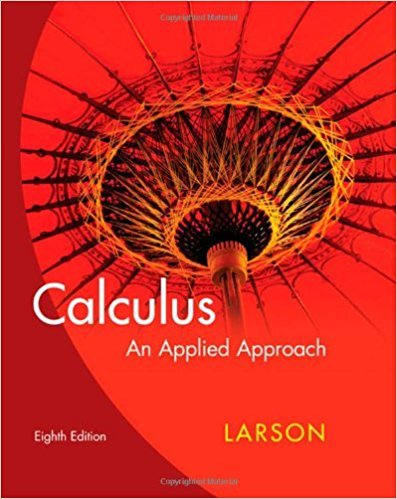×
×

# Solutions for Chapter 9: Probability and Calculus## Full solutions for Calculus: An Applied Approach | 8th Edition

ISBN: 9780618958252Solutions for Chapter 9: Probability and Calculus

Solutions for Chapter 9
4 5 0 250 Reviews
31
5
##### ISBN: 9780618958252

Calculus: An Applied Approach was written by and is associated to the ISBN: 9780618958252. This expansive textbook survival guide covers the following chapters and their solutions. Since 52 problems in chapter 9: Probability and Calculus have been answered, more than 22533 students have viewed full step-by-step solutions from this chapter. Chapter 9: Probability and Calculus includes 52 full step-by-step solutions. This textbook survival guide was created for the textbook: Calculus: An Applied Approach , edition: 8.

Key Calculus Terms and definitions covered in this textbook
• Angle of elevation

The acute angle formed by the line of sight (upward) and the horizontal

• Bias

A flaw in the design of a sampling process that systematically causes the sample to differ from the population with respect to the statistic being measured. Undercoverage bias results when the sample systematically excludes one or more segments of the population. Voluntary response bias results when a sample consists only of those who volunteer their responses. Response bias results when the sampling design intentionally or unintentionally influences the responses

• Common logarithm

A logarithm with base 10.

• Conditional probability

The probability of an event A given that an event B has already occurred

• Coterminal angles

Two angles having the same initial side and the same terminal side

• Equivalent equations (inequalities)

Equations (inequalities) that have the same solutions.

• Expanded form of a series

A series written explicitly as a sum of terms (not in summation notation).

• Imaginary axis

See Complex plane.

• Inductive step

See Mathematical induction.

• Magnitude of a vector

The magnitude of <a, b> is 2a2 + b2. The magnitude of <a, b, c> is 2a2 + b2 + c2

• Modulus

See Absolute value of a complex number.

• Newton’s law of cooling

T1t2 = Tm + 1T0 - Tm2e-kt

The graph in three dimensions of a seconddegree equation in three variables.

• Quantitative variable

A variable (in statistics) that takes on numerical values for a characteristic being measured.

• Repeated zeros

Zeros of multiplicity ? 2 (see Multiplicity).

• Resistant measure

A statistical measure that does not change much in response to outliers.

• Richter scale

A logarithmic scale used in measuring the intensity of an earthquake.

• Sample survey

A process for gathering data from a subset of a population, usually through direct questioning.

• Solution of an equation or inequality

A value of the variable (or values of the variables) for which the equation or inequality is true

• Venn diagram

A visualization of the relationships among events within a sample space.

×